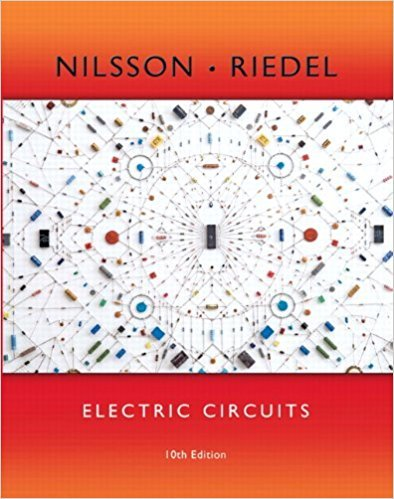×
×

# Solutions for Chapter 17.3: Using Laplace Transforms to Find Fourier Transforms## Full solutions for Electric Circuits | 10th Edition

ISBN: 9780133760033Solutions for Chapter 17.3: Using Laplace Transforms to Find Fourier Transforms

Solutions for Chapter 17.3
4 5 0 325 Reviews
20
2
##### ISBN: 9780133760033

Chapter 17.3: Using Laplace Transforms to Find Fourier Transforms includes 1 full step-by-step solutions. This textbook survival guide was created for the textbook: Electric Circuits, edition: 10. This expansive textbook survival guide covers the following chapters and their solutions. Electric Circuits was written by and is associated to the ISBN: 9780133760033. Since 1 problems in chapter 17.3: Using Laplace Transforms to Find Fourier Transforms have been answered, more than 13334 students have viewed full step-by-step solutions from this chapter.

Key Engineering and Tech Terms and definitions covered in this textbook
×